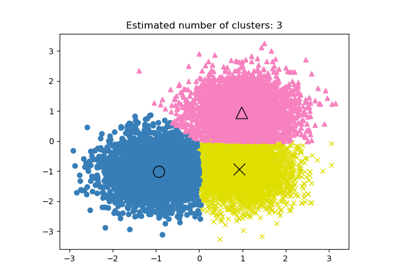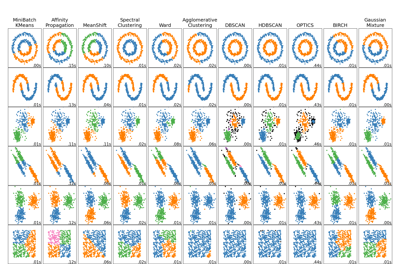# sklearn.cluster.estimate_bandwidth¶

sklearn.cluster.estimate_bandwidth(X, *, quantile=0.3, n_samples=None, random_state=0, n_jobs=None)[source]

Estimate the bandwidth to use with the mean-shift algorithm.

That this function takes time at least quadratic in n_samples. For large datasets, it’s wise to set that parameter to a small value.

Parameters:
Xarray-like of shape (n_samples, n_features)

Input points.

quantilefloat, default=0.3

Should be between [0, 1] 0.5 means that the median of all pairwise distances is used.

n_samplesint, default=None

The number of samples to use. If not given, all samples are used.

random_stateint, RandomState instance, default=None

The generator used to randomly select the samples from input points for bandwidth estimation. Use an int to make the randomness deterministic. See Glossary.

n_jobsint, default=None

The number of parallel jobs to run for neighbors search. None means 1 unless in a joblib.parallel_backend context. -1 means using all processors. See Glossary for more details.

Returns:
bandwidthfloat

The bandwidth parameter.

## Examples using sklearn.cluster.estimate_bandwidth¶A demo of the mean-shift clustering algorithm

A demo of the mean-shift clustering algorithmComparing different clustering algorithms on toy datasets

Comparing different clustering algorithms on toy datasets